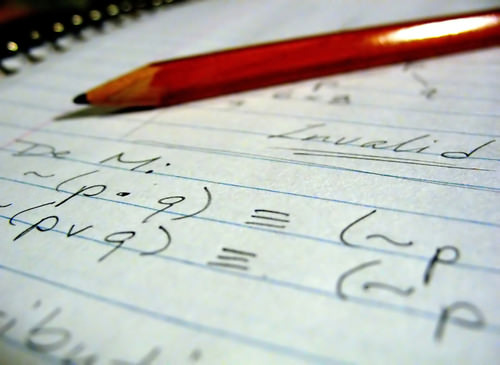# 2.2.1: One-Step Equations and Properties of Equality

•• Contributed by CK12
• CK12

## One-Step Equations and Inverse Operations

What if you were in a math contest and were given the equation x+4=16? Or how about the equation 9x=72? Could you solve each of these equations in one step?Figure $$\PageIndex{1}$$

### One-Step Equations and Inverse Operations

To solve an equation means to write an equivalent equation that has the variable by itself on one side. This is also known as isolating the variable.

Solving One-Step Equations Using Addition or Subtraction

There are four important ideas and properties that you need to solving equations using addition or subtraction. The order of operations, inverse operations, equivalent equations, and the addition property of equality.

You probably have already learned how to simplify an expression using the Order of Operations: Parentheses, Exponents, Multiplication and Division completed in order from left to right, and Addition and Subtraction (also completed from left to right).

Each of these operations has an inverse. Inverse operations “undo” each other when combined and are essential to solving equations. Addition and subtraction are inverses, multiplication and division are inverses, and exponents and roots are inverses.

Equivalent equations are two or more equations with the same solution.

The last tool you need to solve equations using addition or subtraction is the Addition Property of Equality. The Addition Property of Equality allows you to apply the same operation to each side of an equation, in essence: “what you do to one side of an equation you can do to the other.”

The Addition Property of Equality states that for all real numbers a,b, and c:

If a=b, then a+c=b+c.

Because subtraction can be considered “adding a negative,” the Addition Property of Equality also works if you need to subtract the same value from each side of an equation.

#### Let's solve the following equations using the Addition Property of Equality:

1. Solve for y:

16=y−11.

When asked to solve for y, your goal is to write an equivalent equation with the variable y isolated on one side.

Write the original equation: 16=y−11.

The inverse of subtracting 11 is adding 11.

Apply the Addition Property of Equality: 16+11=y−11+11.

Simplify by adding like terms: 27=y.

The solution is y=27.

1. Solve for z:

5=z+12

The inverse of adding 12 is subtracting 12.

Apply the Addition Property of Equality:

5=z+12

5−12=z+12−12

5−12=z

−7=z

The solution is −7=z.

Equations that take one step to isolate the variable are called one-step equations. Such equations can also involve multiplication or division.

#### Solving One-Step Equations Using Multiplication or Division

When solving one-step equations that involve multiplication or division, you will be using inverses and equivalent equations. However, instead of using the Addition Property of Equality, you will use the very similar Multiplication Property of Equality. The Multiplication Property of Equality states that for all real numbers a,b, and c:

If a=b, then a(c)=b(c).

Since division thought of as multiplying by the reciprocal, the Multiplication Property of Equality also applies to dividing both sides of the equation by the same number.

#### Let's solve the following equation using the Multiplication Property of Equality:

Solve for k:−8k=−96.

Because −8k=−8×k, the inverse operation of multiplication is division. Therefore, we must cancel multiplication by applying the Multiplication Property of Equality.

Write the original equation: −8k=−96.

The inverse of multiplying by 8 is dividing by 8.

Apply the Multiplication Property of Equality: −8k÷−8=−96÷−8.

The solution is k=12.

When working with fractions, you must remember: a/b×b/a=1. In other words, in order to cancel a fraction using division, you really must multiply by its reciprocal.

### Examples

Example 2.2.1.1

Earlier, you were asked to solve the equations x+4=16 and 9x=72.

x+4=16

Solution

ince this is an addition problem, we will be applying the Addition Property of Equality. The inverse of adding 4 is subtracting 4.

x+4=16

x+4−4=16−4

x=12

The solution for x+4=16 is x=12.

9x=72

Since this is a multiplication problem, we will be applying the Addition Property of Equality. The inverse of multiplying by 9 is dividing by 9.

9x=72

9x÷9=72÷9

x=8

The solution for 9x=72 is x=8.

Example 2.2.1.2

Solve 18⋅x=1.5.

Solution

The variable x is being multiplied by one-eighth. Instead of dividing two fractions, we multiply by the reciprocal of 1/8, which is 8.

8(1/8⋅x=8(1.5)

x=12

### Review

Solve for the given variable.

1. x+11=7
2. x−1.1=3.2
3. 7x=21
4. 4x=1
5. 5x/12=2/3
6. x+5/2=2/3
7. x−5/6=3/8
8. 0.01x=11
9. q−13=−13
10. z+1.1=3.0001
11. 21s=3
12. t+1/2=1/3
13. 7f/11=7/11
14. 3/4=−1/2⋅y
15. 6r=3/8
16. 9b/16=3/8

### Vocabulary

Term Definition
Addition Property of Equality For all real numbers a,b, and c: If a=b, then a+c=b+c.
equivalent equation By applying the same inverse operations to each side of an equation, you create an equivalent equation. Equivalent equations are two or more equations having the same solution.
inverse operation Each of these operations has an inverse. Inverse operations undo each other when combined.
Multiplication Property of Equality For all real numbers a,b, and c: If a=b, then a(c)=b(c).
one-step equations Equations that take one step to isolate the variable. Such equations can also involve multiplication or division.
solving equations To solve an equation means to write an equivalent equation that has the variable by itself on one side. This is also known as isolating the variable.
constant A constant is a value that does not change. In Algebra, this is a number such as 3, 12, 342, etc., as opposed to a variable such as x, y or a.
Equation An equation is a mathematical sentence that describes two equal quantities. Equations contain equals signs.
Numerical Coefficient In mathematical expressions, the numerical coefficients are the numbers associated with the variables. For example, in the expression 4x, 4 is the numerical coefficient and x is the variable.
Variable A variable is a symbol used to represent an unknown or changing quantity. The most common variables are a, b, x, y, m, and n.